# Limits and Continuity Solutions Exercise 8(e) Inter

Intermeduate Mathematics 1B textbook Chapter 8 Limits and Continuity Exercise 8(e)  solutions are given.

First you sytudy the textbook lesson very well. Then observe the example problems and solutions given in the textbook.

The keypoints in the lesson are very important.

Observe the solutions given below and try them in your own method.

You can also see

Inter Maths 1A textbook solutions

Inter Maths 1B textbook solutions

Inter Maths IIA textbook solutions

Inter Maths IIB textbook solutions

Limits and Continuity

Exercise 8(a)

Exercise 8(b)

Exetcise 8(c)

Exetcise 8(d)

Exercise 8(e)

M

## Maths 1b solutions Inter Limits and Continuity exercise 8(e)

Limits and Continuity

Exercise 8(e)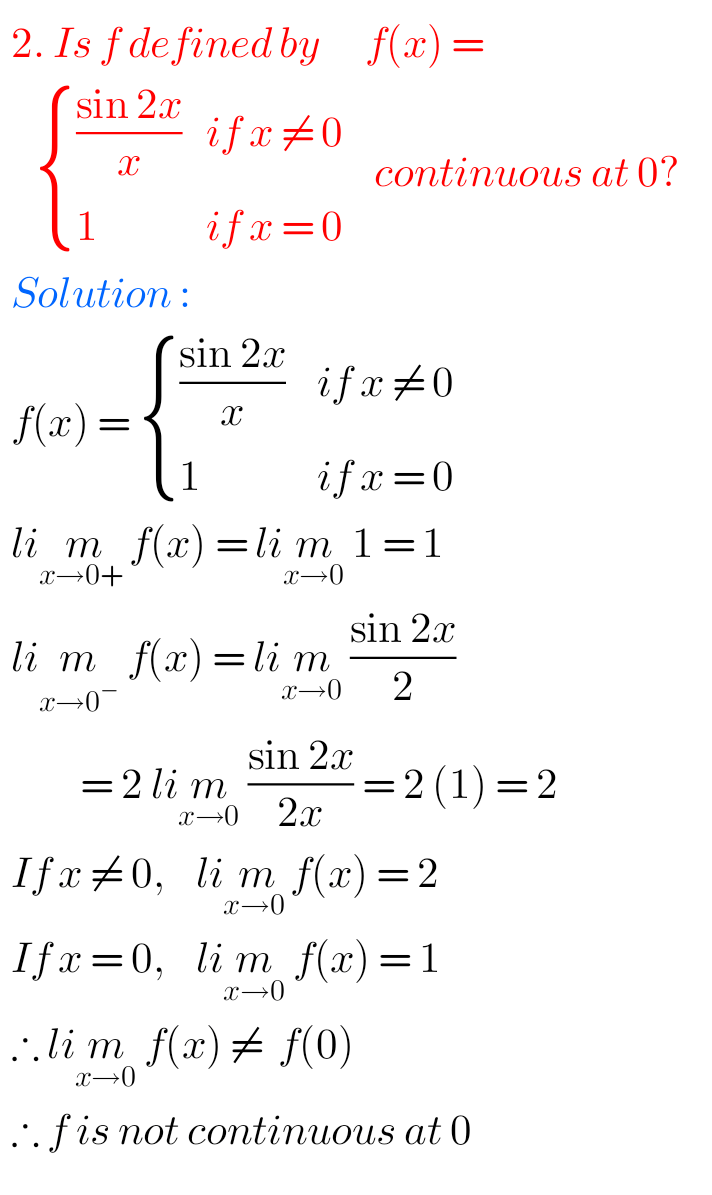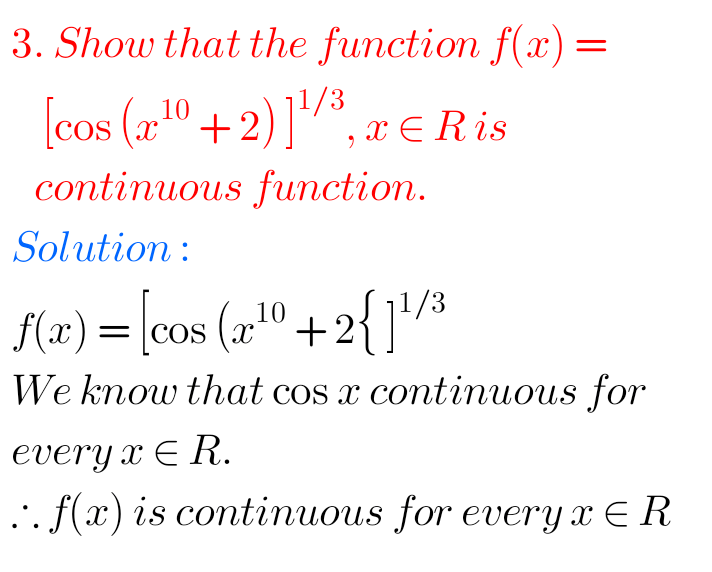## Solutions for Limits and Continuity Exercise 8(e) Inter Maths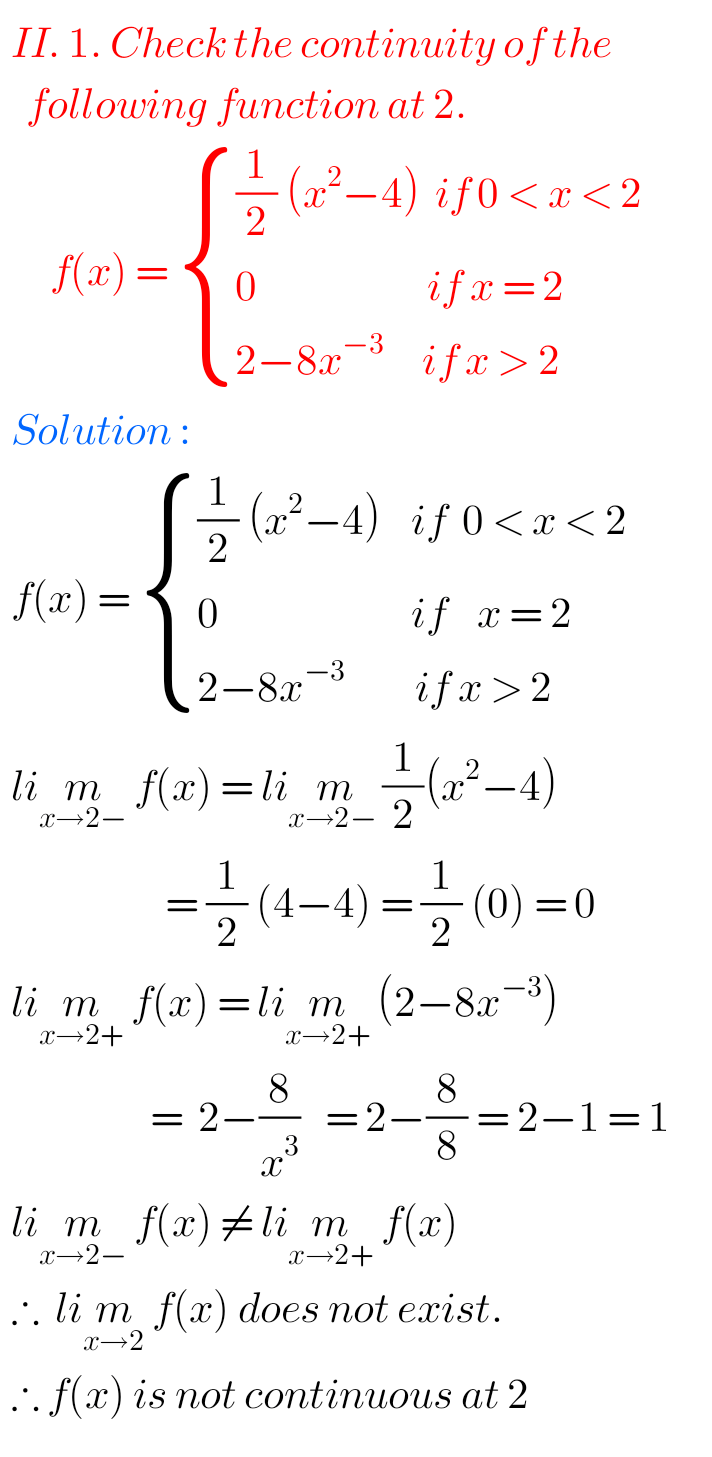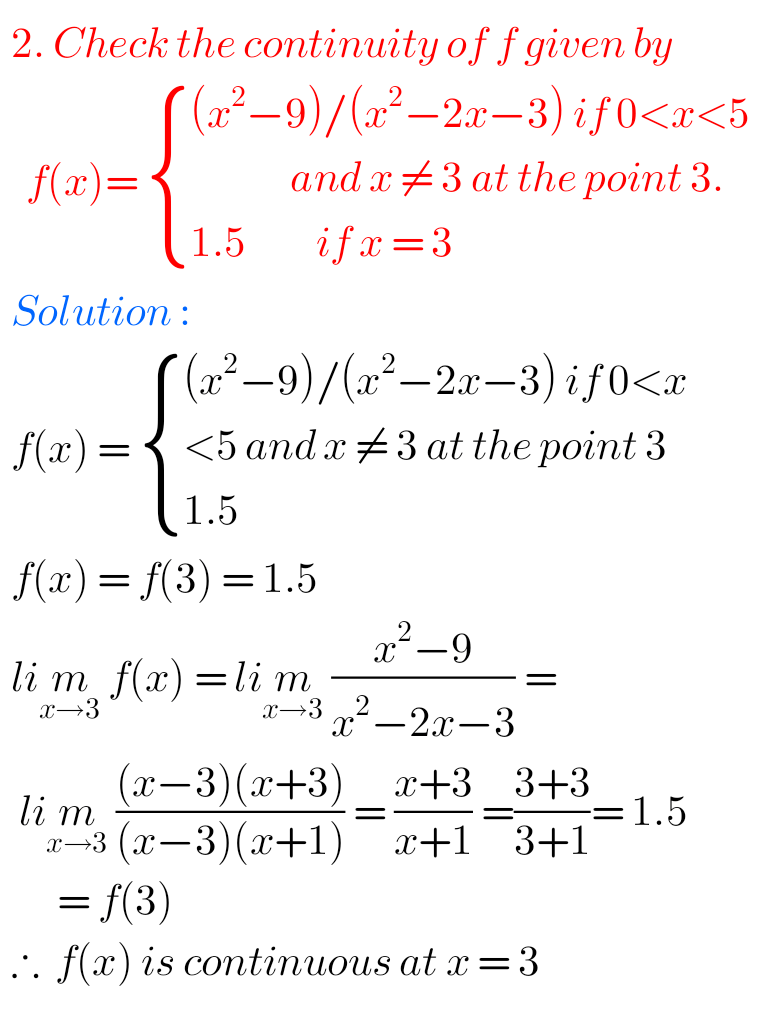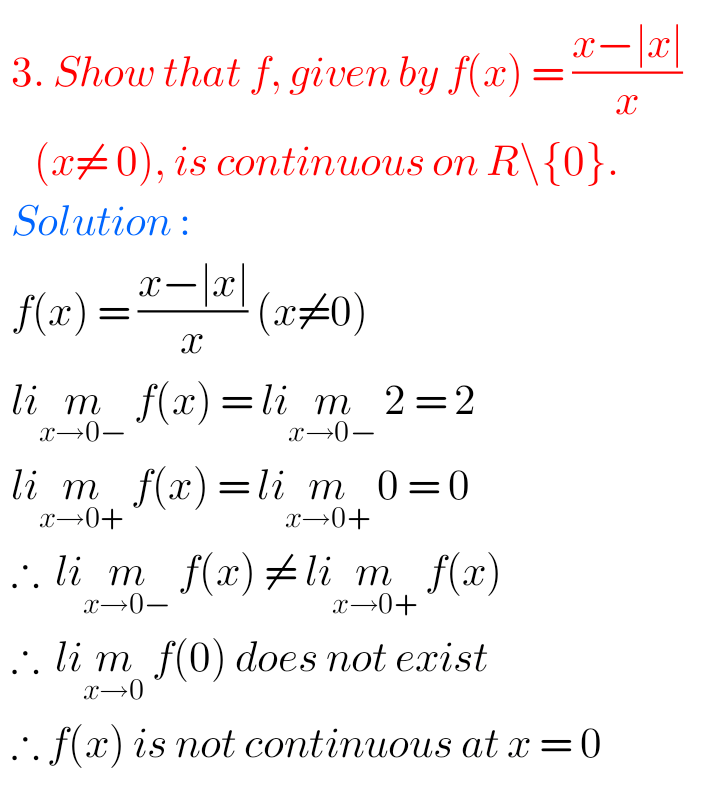## Exercise 8(e) Chapter 8 Limits and continuity Maths 1b Inter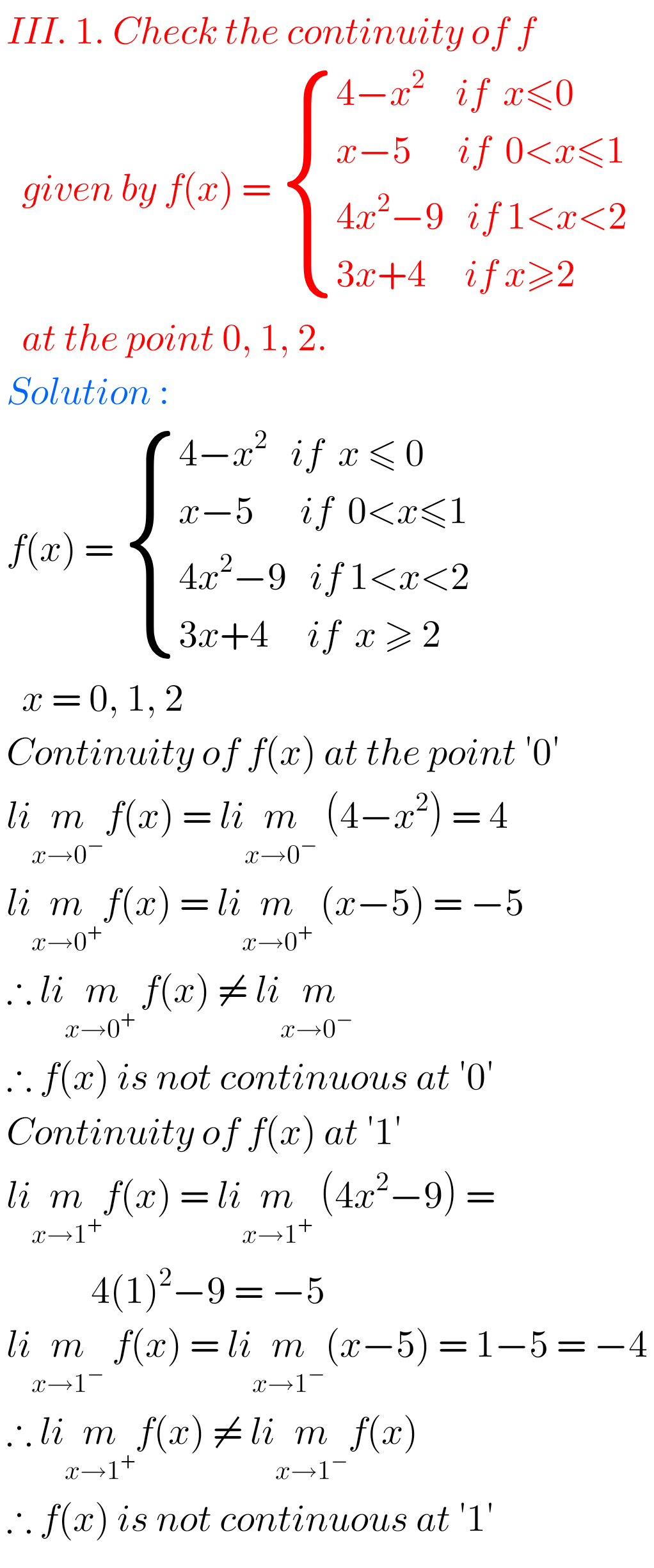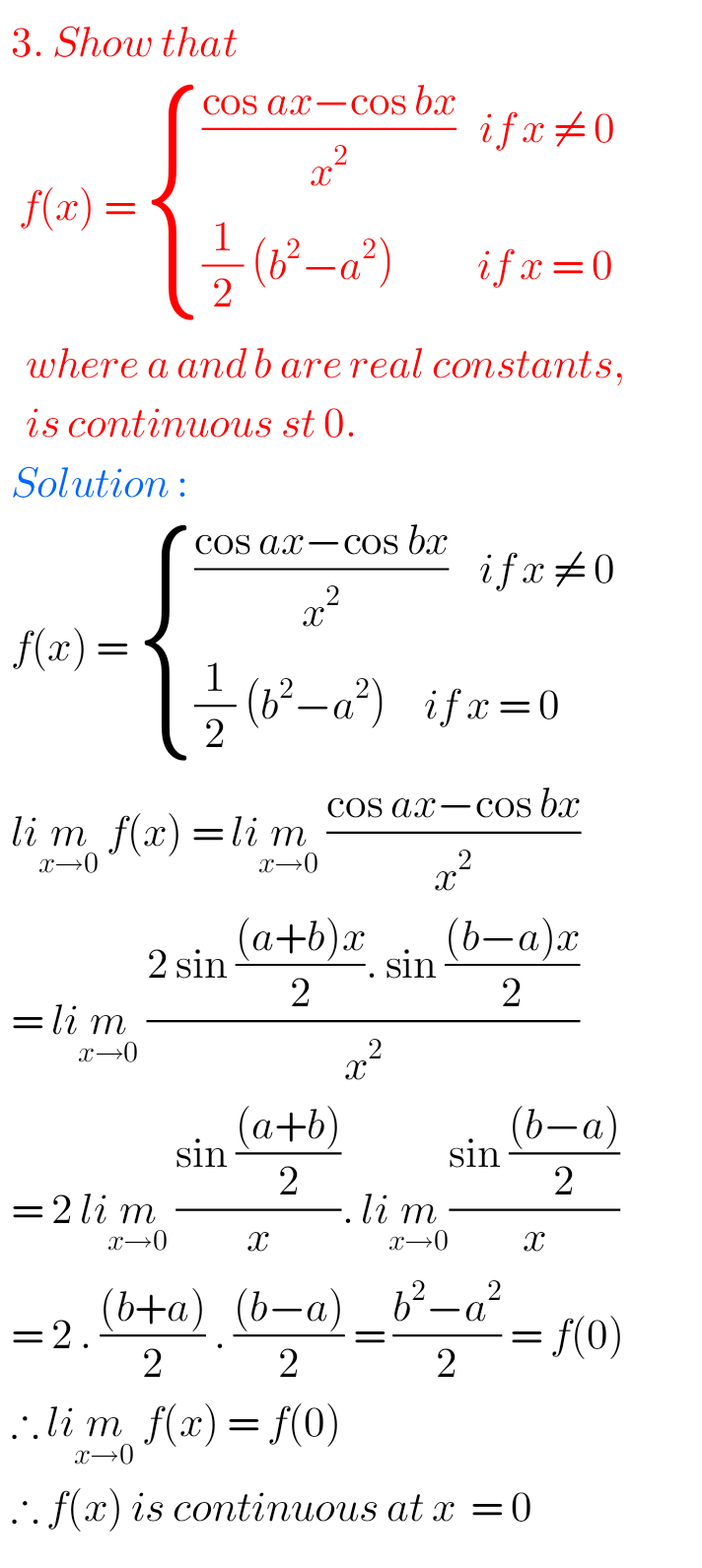M

Note : Observe the solutions and try them in your own method.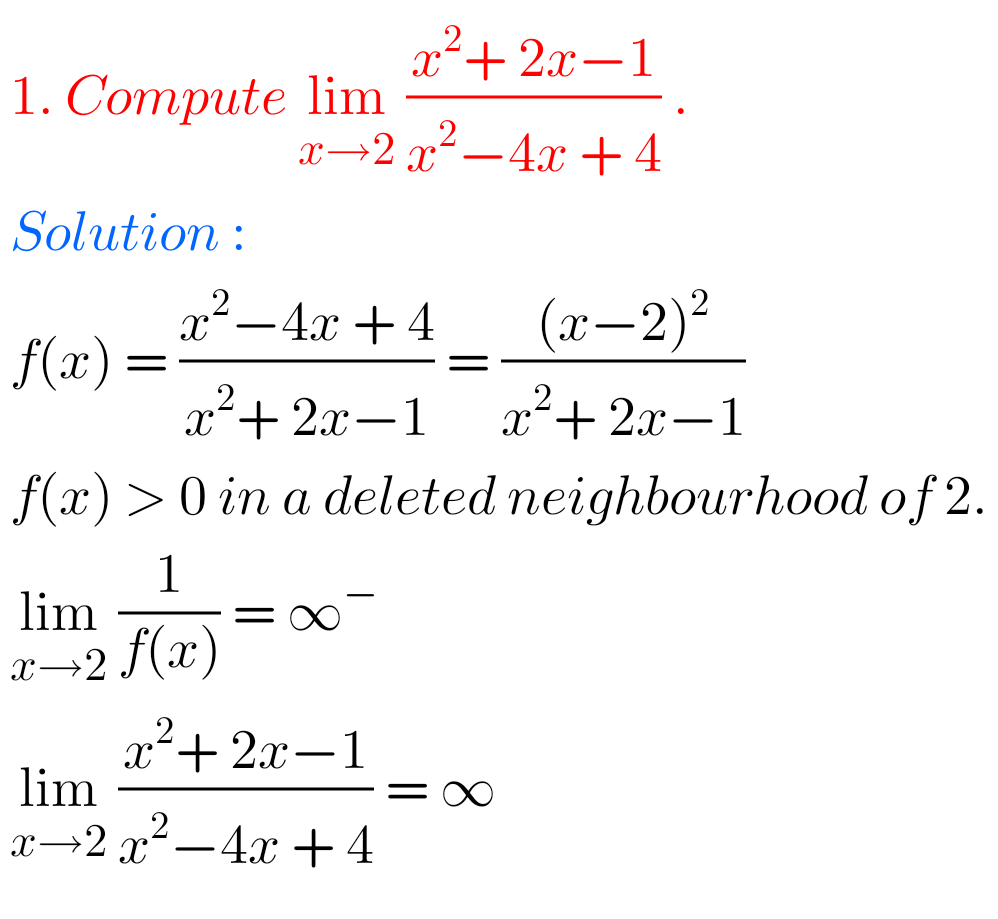SSC Maths textbook solutions class 10

Ncert maths class 6 chapter 14 solutions

Nios maths 311 book 1 Sets 1.1 solutions